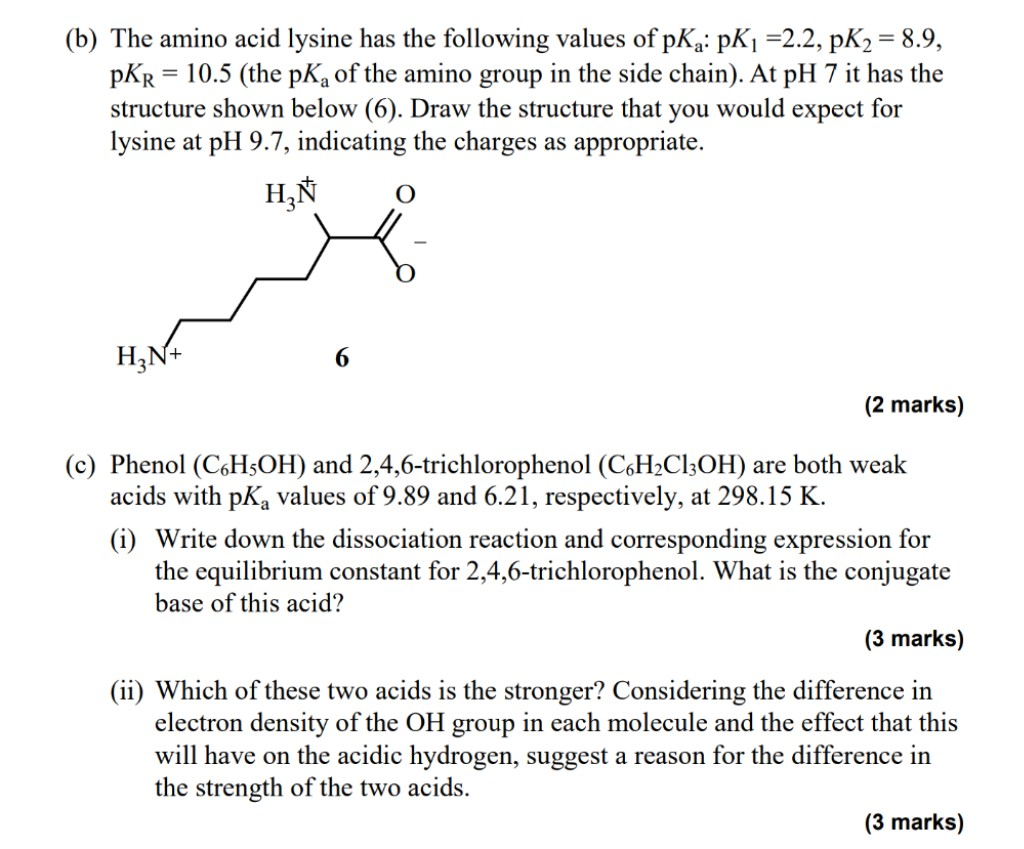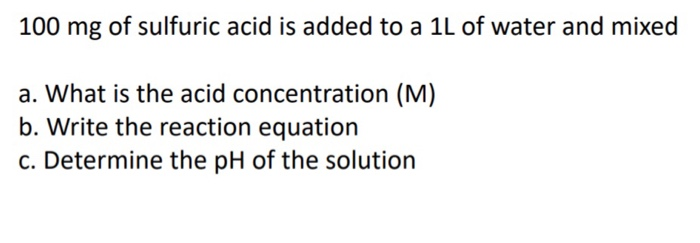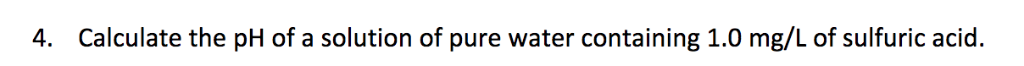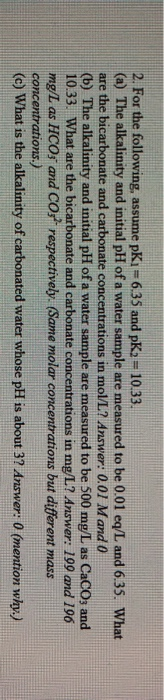Question

A stream has a sulfuric acid concentration of 5000 mg/L. Assume pK1 = -3 and pK2 = 1.99. What is the expected pH of the stream?

We need at least 10 more requests to produce the answer.

0 / 10 have requested this problem solution

The more requests, the faster the answer.

All students who have requested the answer will be notified once they are available.

#### Earn Coins

Coins can be redeemed for fabulous gifts.

Similar Homework Help Questions
• ### The amino acid Prolinehas a pk1 of 1.99, pk2 of 10.60. The amino acid Serine has...

The amino acid Prolinehas a pk1 of 1.99, pk2 of 10.60. The amino acid Serine has pk1 of 2.21 and a of pk2 of 9.15. If these two amino acids bind together by peptide bond creating the peptide sequence Pro-Ser . What will be the overall charge of this peptide at the physiological pH of 7?

• ### Given that pK1 = 3.0 and pK2 = 8.0 for an amino acid X. Total concentration...

Given that pK1 = 3.0 and pK2 = 8.0 for an amino acid X. Total concentration of amino acid X in solution is 10 mM. Calculate the concentrations of protonated form (P), dipolar form (Z) and de-protonated form (D) of amino acid X at pH 2, and 10.

• ### The amino acid Tyrosine (H3Tyr) has pK1 = 2.41, pK2 = 8.67, and pK3 = 11.01....

The amino acid Tyrosine (H3Tyr) has pK1 = 2.41, pK2 = 8.67, and pK3 = 11.01. (a) What is the principal form of Tyrosine at pH 9.5 and why? (b) What is the second prominent species at pH 6.5 and pH 10.8? *Please show all work! Thank you!!

• ### #1) a) The pK1, pK2, and pKR for the amino acid histidine are 1.8, 9.3, and...

#1) a) The pK1, pK2, and pKR for the amino acid histidine are 1.8, 9.3, and 6.0, respectively. At pH 4.0 would be charged predominantly as follows: A a-carboxylate 0, a-amino +1, imidazole +1, net charge +2 B a-carboxylate +1, a-amino +1, imidazole −1, net charge +1 C a-carboxylate −1, a-amino +1, imidazole 0, net charge 0 D a-carboxylate +1, a-amino 0, imidazole −1, net charge 0 E a-carboxylate −1, a-amino +1, imidazole +1, net charge +1 b) The pK1,...

• ### (b) The amino acid lysine has the following values of pK : PK1 =2.2, pK2 = 8.9, PKR = 10.5 (the pK, of the amino gro...(b) The amino acid lysine has the following values of pK : PK1 =2.2, pK2 = 8.9, PKR = 10.5 (the pK, of the amino group in the side chain). At pH 7 it has the structure shown below (6). Draw the structure that you would expect for lysine at pH 9.7, indicating the charges as appropriate. HZN 0 HINA (2 marks) (c) Phenol (C6H5OH) and 2,4,6-trichlorophenol (C6H2C13OH) are both weak acids with pK, values of 9.89 and 6.21, respectively,...

• ### 100 mg of sulfuric acid is added to a 1L of water and mixed a. What is the acid concentration (M) b. Write the reac...100 mg of sulfuric acid is added to a 1L of water and mixed a. What is the acid concentration (M) b. Write the reaction equation c. Determine the pH of the solution

• ### 4. Calculate the pH of a solution of pure water containing 1.0 mg/L of sulfuric acid.4. Calculate the pH of a solution of pure water containing 1.0 mg/L of sulfuric acid.

• ### A stream during late winter has a temperature of 5 °C and 5 mg DO/L. a)...

A stream during late winter has a temperature of 5 °C and 5 mg DO/L. a) What is the percent saturation of DO? There is a spell of hot weather and the water temperature increases to 15 °C (at the same 5 mg DO/L). b) What is the new percent DO saturation? c) Even though the DO concentration was the same in terms of mg/L, why do these percent saturation values differ? d) What does this mean qualitatively for aquatic...

• ### need HW help thx! 2. For the following, assume pKi = 6.35 and pK2 10.33. (a) The alkalinity and initial pH of a wate...need HW help thx! 2. For the following, assume pKi = 6.35 and pK2 10.33. (a) The alkalinity and initial pH of a water sample are measured to be 0.01 eq/L and 6.35. What are the bicarbonate and carbonate concentrations in mol/L? Answer: 0.01 Mand 0 (b) The alkalinity and initial pH of a water sample are measured to be 500 mg/L as CaCO3 and 10.33. What are the bicarbonate and carbonate concentrations in mg/L? Answer: 199 and 196 mg/L...

• ### What is the concentration of sulfuric acid in the following scenario?

A #10.0*mL# volume of sulfuric acid, that had been prediluted TENFOLD, was titrated by a #67.02*mL# volume of #NaOH(aq)# of #6.000*mol*L^-1# concentration. What is #[H_2SO_4]#?